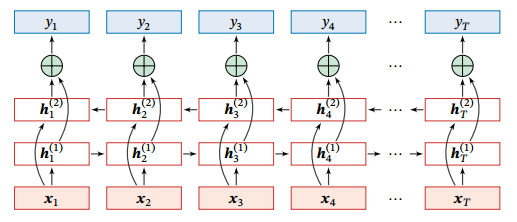# RNN

【注】参考自邱锡鹏的《神经网络与深度学习》。

## 1. 简介

• RNN 能够用于处理时序数据的神经网络，被广泛应用于语音识别、语言模型以及自然语言生成等任务上。

• 理论上，前馈神经网络可以模拟任何连续函数，而循环神经网络可以模拟任何程序。

## 2. 给网络增加记忆能力

### 2.1 延时神经网络

• 延时神经网络（TDNN）是在前馈网络中的非输出层都添加一个延时器，记录神经元的最近几次活性值。

• 在第 $t$ 个时刻，第 $l$ 层神经元的活性值依赖于第 $l - 1$ 层神经元的最近 $K$ 个时刻的活性值，即

$\begin{array}{c} \boldsymbol{h}_t^{(l)} = f(\boldsymbol{h}_t^{(l-1)}, \boldsymbol{h}_{t-1}^{(l-1)}, \cdots, \boldsymbol{h}_{t-K}^{(l-1)}) \end{array}$

### 2.2 有外部输入的非线性自回归模型

• 自回归模型（AR）是统计学上常用的一类时间序列模型，用一个变量 $\boldsymbol{y}_t$ 的历史信息来预测自己：

$\begin{array}{c} \boldsymbol{y}_t = w_0 + \sum_{k=1}^K w_k \boldsymbol{y}_{t-k} + \epsilon_t \end{array}$

• 有外部输入的非线性自回归模型（NARX）是自回归模型的扩展，在每个时刻 $t$ 都有一个外部输入 $\boldsymbol{x}_t$，产生一个输出 $\boldsymbol{y}_t$ 。NARX 通过一个延时器记录最近 $K_x$ 次的外部输入和最近 $K_y$ 次的输出，第 $t$ 个时刻的输出 $\boldsymbol{y}_t$ 为：

$\begin{array}{c} \boldsymbol{y}_t = f(\boldsymbol{x}_t, \boldsymbol{x}_{t-1}, \cdots, \boldsymbol{x}_{t-K_x}, \boldsymbol{y}_{t-1}, \boldsymbol{y}_{t-2}, \cdots, \boldsymbol{y}_{t-K_y}) \end{array}$

### 2.3 循环神经网络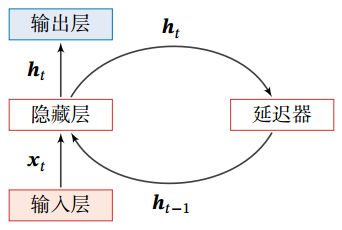$\begin{array}{c} \boldsymbol{h}_t = f(\boldsymbol{h}_{t-1}, \boldsymbol{x}_t) \end{array}$

## 3. 简单循环网络

$\begin{array}{c} \boldsymbol{z}_t = \boldsymbol{U}\boldsymbol{h}_{t-1} + \boldsymbol{W} \boldsymbol{x}_t + \boldsymbol{b} \\ \boldsymbol{h}_t = f(\boldsymbol{z}_t) \end{array}$

• 如果将每个时刻的状态都看作前馈神经网络的一层，循环神经网络可以看作在时间维度上权值共享的神经网络。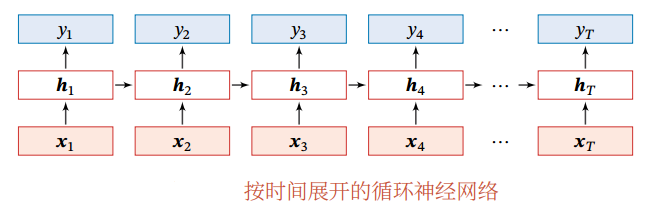### 3.1 循环神经网络的通用近似定理

$\begin{array}{c} \boldsymbol{s}_t = g(\boldsymbol{s}_{t-1}, \boldsymbol{x}_t) \\ \boldsymbol{y}_t = o(\boldsymbol{s}_t) \end{array}$



#### 证明：

$\begin{array}{c} \boldsymbol{h}_t = f(\boldsymbol{U} \boldsymbol{h}_{t-1} + \boldsymbol{W} \boldsymbol{x}_t + \boldsymbol{b}) \\ \boldsymbol{y}_t = \boldsymbol{V} \boldsymbol{h}_t \end{array}$



## 4. 循环神经网络应用分类

### 4.1 序列到类别模式

• 假设一个样本 $\boldsymbol{x}_{1:T} = (\boldsymbol{x}_1, \cdots, \boldsymbol{x}_T)$ 为一个长度为 $T$ 的序列，输出为一个类别 $y \in \{1,\cdots,C\}$，则可以将样本 $\boldsymbol{x}$ 按不同时刻输入到循环神经网络中，并得到不同时刻的隐藏状态 $\boldsymbol{h}_1, \cdots, \boldsymbol{h}_T$

• 我们可以将 $\boldsymbol{h}_T$ 看作整个序列的最终表示输入给分类器 $g(\cdot)$ 进行分类：

$\begin{array}{c} \hat{y} = g(\boldsymbol{h}_T) \end{array}$

$\begin{array}{c} \hat{y} = g(\frac{1}{T} \sum_{t=1}^T \boldsymbol{h}_t) \end{array}$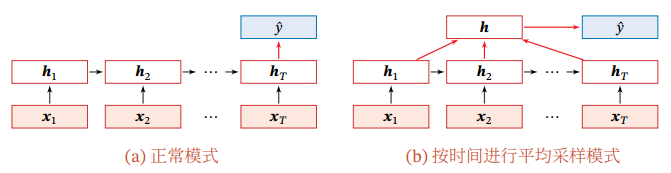### 4.2 同步的序列到序列模式

• 在同步的序列到序列模式中，输入为一个长度为 $T$ 的序列 $\boldsymbol{x}_{1:T} = (\boldsymbol{x}_1, \cdots, \boldsymbol{x}_T)$，输出为序列 $y_{1:T} = (y_1,\cdots,y_T)$。样本 $\boldsymbol{x}$ 按不同时刻输入到循环神经网络中，并得到不同时刻的隐状态 $\boldsymbol{h}_1, \cdots, \boldsymbol{h}_T$ 。每个时刻的隐状态 $\boldsymbol{h}_t$ 代表了当前时刻和历史的信息，并输入给分类器 $g(\cdot)$ 得到当前时刻的标签 $\hat{y}_t$

$\begin{array}{c} \hat{y}_t = g(\boldsymbol{h}_t), \quad \forall t \in [1, T] \end{array}$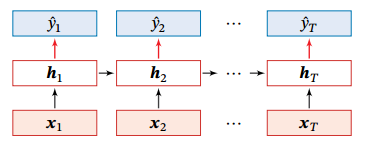### 4.3 异步的序列到序列模式

• 在异步的序列到序列模式中，输入为一个长度为 $T$ 的序列 $\boldsymbol{x}_{1:T} = (\boldsymbol{x}_1, \cdots, \boldsymbol{x}_T)$，输出为长度为 $M$ 的序列 $y_{1:M} = (y_1,\cdots,y_M)$

• 异步的序列到序列模式一般通过先编码后解码的方式来实现。先将样本 $\boldsymbol{x}$ 按不同时刻输入到一个循环神经网路（编码器）中，并得到其编码 $\boldsymbol{h}_T$；然后再使用另一个循环神经网络（解码器）得到输出序列 $\hat{y}_{1:M}$

• 为了建立输出序列之间的依赖关系，在解码器中通常使用非线性的自回归模型。令 $f(\cdot)$$f(\cdot)$ 分别为用作编码器和解码器的循环神经网络，则编码器-解码器模型可以写为：

$\begin{array}{c} \boldsymbol{h}_t = f_1(\boldsymbol{h}_{t-1}, \boldsymbol{x}_t), \quad \forall t \in [1,T] \\ \boldsymbol{h}_{T+t} = f_2(\boldsymbol{h}_{T+t-1}, \hat{y}_{t-1}), \quad \forall t \in [1,M] \\ \hat{y}_t = g(\boldsymbol{h}_{T+t}) \quad \forall t \in [1, M] \end{array}$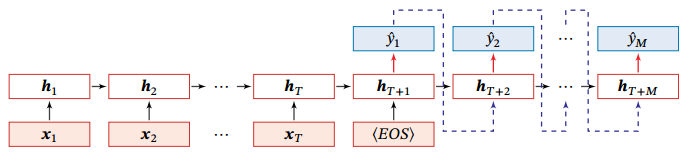## 5. 计算梯度

• 定义时刻 $t$ 的损失函数为：

$\begin{array}{c} \mathcal{L}_t = \mathcal{L}(y_t, g(\boldsymbol{h}_t)) \end{array}$

$\begin{array}{c} \mathcal{L} = \sum_{t=1}^T \mathcal{L}_t \end{array}$

• 整个序列的损失函数 $\mathcal{L}$ 关于参数 $\boldsymbol{U}, \boldsymbol{W}, \boldsymbol{b}$ 的梯度为：

$\begin{array}{c} \frac{\partial \mathcal{L}}{\partial \boldsymbol{U}} = \sum_{t=1}^T \frac{\partial \mathcal{L}_t}{\partial \boldsymbol{U}} \\ \frac{\partial \mathcal{L}}{\partial \boldsymbol{W}} = \sum_{t=1}^T \frac{\partial \mathcal{L}_t}{\partial \boldsymbol{W}} \\ \frac{\partial \mathcal{L}}{\partial \boldsymbol{b}} = \sum_{t=1}^T \frac{\partial \mathcal{L}_t}{\partial \boldsymbol{b}} \end{array}$

### 5.1 随时间反向传播算法（BPTT）

BPTT 算法将循环神经网络看作一个展开的多层前馈网络，其中「每一层」对应循环网络中的「每个时刻」；这样，循环神经网络就可以按照前馈网络中的反向传播算法计算参数梯度。

• 计算偏导数 $\frac{\partial \mathcal{L}_t}{\partial \boldsymbol{U}}, \frac{\partial \mathcal{L}_t}{\partial \boldsymbol{W}}, \frac{\partial \mathcal{L}_t}{\partial \boldsymbol{b}}$

$\begin{array}{c} \frac{\partial \mathcal{L}_t}{\partial u_{ij}} = \sum_{k=1}^t \frac{\partial^+ \boldsymbol{z}_k}{\partial u_{ij}} \frac{\partial \mathcal{L}_t}{\partial \boldsymbol{z}_k} \end{array}$

$\begin{array}{c} \frac{\partial^+ \boldsymbol{z}_k}{\partial u_{ij}} = [0, \cdots, [\boldsymbol{h}_{k-1}], \cdots, 0] \triangleq \mathbb{I}_i([\boldsymbol{h}_{k-1}]_j) \end{array}$

$\begin{array}{c} \delta_{t,k} = \frac{\partial \mathcal{L}_t}{\partial \boldsymbol{z}_k} = \frac{\partial \boldsymbol{h}_k}{\partial \boldsymbol{z}_k} \frac{\partial \boldsymbol{z}_{k+1}}{\partial \boldsymbol{h}_k} \frac{\partial \mathcal{L}_t}{\partial \boldsymbol{z}_{k+1}} = \mathrm{diag}(f^{'}(\boldsymbol{z}_k)) \boldsymbol{U}^T \delta_{t,k+1} \end{array}$

$\begin{array}{c} \frac{\partial \mathcal{L}_t}{\partial u_{ij}} = \sum_{k=1}^t [\delta_{t,k}]_i [\boldsymbol{h}_{k-1}]_j \end{array}$

$\begin{array}{c} \frac{\partial \mathcal{L}_t}{\partial \boldsymbol{U}} = \sum_{k=1}^t \delta_{t,k} \boldsymbol{h}_{k-1}^T \end{array}$

$\begin{array}{c} \frac{\partial \mathcal{L}}{\partial \boldsymbol{U}} = \sum_{t=1}^T \sum_{k=1}^t \delta_{t,k} \boldsymbol{h}_{k-1}^T \\ \frac{\partial \mathcal{L}}{\partial \boldsymbol{W}} = \sum_{t=1}^T \sum_{k=1}^t \delta_{t,k} \boldsymbol{x}_k^T \\ \frac{\partial \mathcal{L}}{\partial \boldsymbol{b}} = \sum_{t=1}^T \sum_{k=1}^t \delta_{t,k} \end{array}$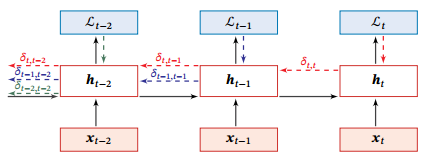### 5.2 实时循环学习算法（RTRL）

RTRL 通过前向传播的方式来计算梯度。假设循环神经网络中第 $t+1$ 时刻的状态 $\boldsymbol{h}_{t+1}$

$\begin{array}{c} \boldsymbol{h}_{t+1} = f(\boldsymbol{z}_{t+1}) = f(\boldsymbol{U}\boldsymbol{h}_t + \boldsymbol{W}\boldsymbol{x}_{t+1} + \boldsymbol{b}) \end{array}$

$\begin{array}{c} \frac{\partial \boldsymbol{h}_{t+1}}{\partial u_{ij}} = (\frac{\partial^+ \boldsymbol{z}_{t+1}}{\partial u_{ij}} + \frac{\partial \boldsymbol{h}_t}{\partial u_{ij}}\boldsymbol{U}^T) \mathrm{diag}(f^{'}(\boldsymbol{z}_{t+1})) = (\mathbb{I}_i([\boldsymbol{h}_t]_j) + \frac{\partial \boldsymbol{h}_t}{\partial u_{ij}}\boldsymbol{U}^T) \odot (f^{'}(\boldsymbol{z}_{t+1}))^T \end{array}$

$\begin{array}{c} \frac{\partial \mathcal{L}_t}{\partial u_{ij}} = \frac{\partial \boldsymbol{h}_t}{\partial u_{ij}} \frac{\partial \mathcal{L}_t}{\partial \boldsymbol{h}_t} \\ \frac{\partial \mathcal{L}_t}{\partial w_{ij}} = \frac{\partial \boldsymbol{h}_t}{\partial w_{ij}} \frac{\partial \mathcal{L}_t}{\partial \boldsymbol{h}_t} \\ \frac{\partial \mathcal{L}_t}{\partial b_i} = \frac{\partial \boldsymbol{h}_t}{\partial b_i} \frac{\partial \mathcal{L}_t}{\partial \boldsymbol{h}_t} \end{array}$

## 6. 基于门控的循环神经网络

### 6.1 长短期记忆网络

• 遗忘门 $\boldsymbol{F}_t$：控制上一个时刻的内部状态 $\boldsymbol{C}_{t-1}$ 需要遗忘多少信息。

• 输入门 $\boldsymbol{I}_t$：控制当前时刻的候选状态 $\tilde{\boldsymbol{C}}_t$ 有多少信息需要保存。

• 输出门 $\boldsymbol{O}_t$：控制当前时刻的内部状态 $\boldsymbol{C}_t$ 有多少信息需要输出给外部状态 $\boldsymbol{H}_t$

LSTM 的循环单元结构如下：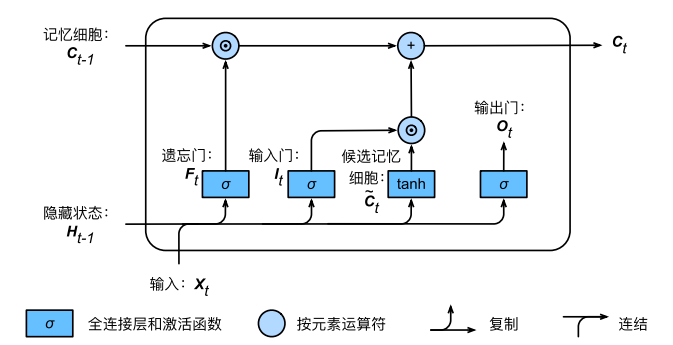### 6.2 门控循环单元网络

• 重置门 $\boldsymbol{R}_t$：控制了上⼀时间步的隐藏状态如何流⼊当前时间步的候选隐藏状态。重置⻔有助于捕捉时间序列⾥短期的依赖关系

• 更新门 $\boldsymbol{Z}_t$：控制隐藏状态应该如何被包含当前时间步信息的候选隐藏状态所更新。更新⻔有助于捕捉时间序列⾥⻓期的依赖关系

GRU 循环单元结构如下：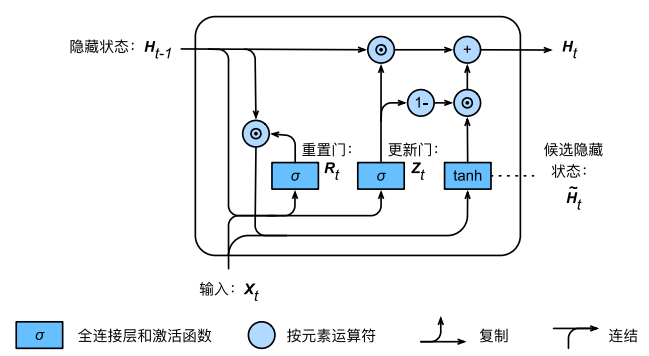## 7. 深层循环神经网络

### 7.1 堆叠循环神经网络

$\begin{array}{c} \boldsymbol{h}_t^{(l)} = f(\boldsymbol{U}^{(l)} \boldsymbol{h}_{t-1}^{(l)} + \boldsymbol{W}^{(l)} \boldsymbol{h}_t^{(l-1)} + \boldsymbol{b}^{(l)}) \end{array}$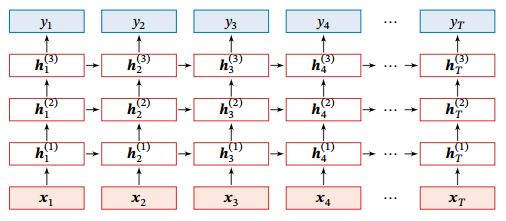### 7.2 双向循环神经网络

$\begin{array}{c} \boldsymbol{h}_t^{(1)} = f(\boldsymbol{U}^{(1)} \boldsymbol{h}_{t-1}^{(1)} + \boldsymbol{W}^{(1)} \boldsymbol{x}_t + \boldsymbol{b}^{(1)}) \\ \boldsymbol{h}_t^{(2)} = f(\boldsymbol{U}^{(2)} \boldsymbol{h}_{t+1}^{(2)} + \boldsymbol{W}^{(2)} \boldsymbol{x}_t + \boldsymbol{b}^{(2)}) \\ \boldsymbol{h}_t = \boldsymbol{h}_t^{(1)} \oplus \boldsymbol{h}_t^{(2)} \end{array}$